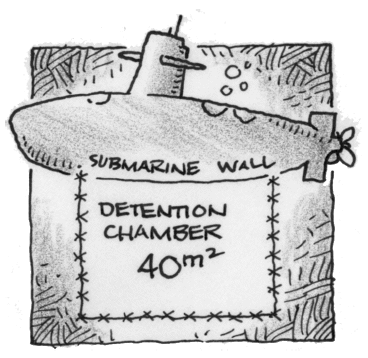### Home > CALC3RD > Chapter Ch5 > Lesson 5.1.4 > Problem5-39

5-39.YELLOW SUBMARINE, Part One

Ring O. Star is sick and tired of chasing blue meanies around the inside of his yellow submarine, so he decides to build a special detention chamber to hold the ones he catches. The first design he thinks of is a rectangular chamber with sides made of razor-sharp barbed wire. To save money, he decides to build the chamber up against a submarine wall—that way only three sides needed to be made out of barbed wire. He estimates the detention chamber needs to have $40$ square meters of area. What dimensions should the chamber have so that Mr. Star can buy the least amount of barbed wire? Homework Help ✎

You are being asked to 'minimize' perimeter. Did you recognize that this is an optimization problem?
The first thing you will do is build a geometric equation that represents the situation. Then you will apply Calculus.

What do we know?

$\text{Area}: bh=40\rightarrow b=\frac{40}{h}$

$\text{Perimeter (there are only three sides)}: P=b+2h=\frac{40}{h}+2h$

Now that we have an equation, $P\left(h\right)$, let's find it's minimum value.

$P(h)=\frac{40}{h}+2h$

$P^\prime(h)$ = ___________________

To find the $h$-value of the max and min, set $P^\prime(h)=0$, and solve for $h$.
Then, for a complete answer, don't forget to find the corresponding value of $b$.